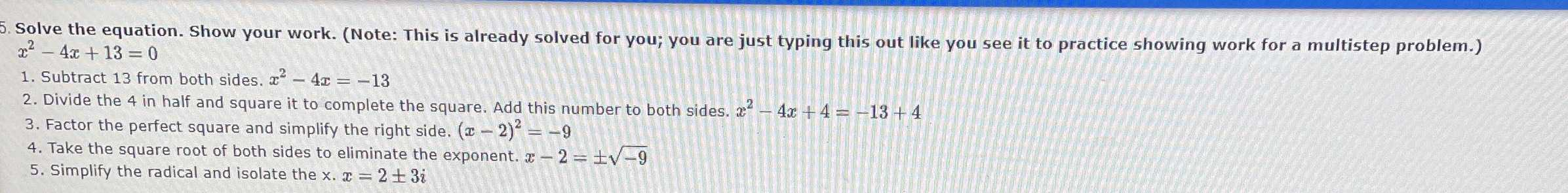### ¿Todavía tienes preguntas de matemáticas?

Pregunte a nuestros tutores expertos
Algebra
Pregunta5. Solve the equation. Show your work. (Note: This is already solved for you; you are just typing this out like you see it to practice showing work for a multistep problem.) $$x ^ { 2 } - 4 x + 13 = 0$$

1. Subtract $$13$$ from both sides. $$x ^ { 2 } - 4 x = - 13$$

2. Divide the $$4$$ in half and square it to complete the square. Add this number to both sides. $$x ^ { 2 } - 4 x + 4 = - 13 + 4$$

3. Factor the perfect square and simplify the right side. $$( x - 2 ) ^ { 2 } = - 9$$

4. Take the square root of both sides to eliminate the exponent. $$x - 2 = \pm \sqrt { - 9 }$$

5. Simplify the radical and isolate the $$x . x = 2 \pm 3 i$$

$$2+ 3i,2- 3i$$